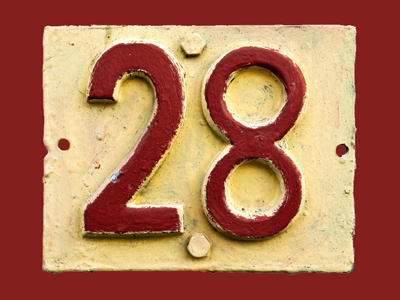2 x 14 = 28.

# The 14 Times Table

If you want to do well in maths it's a good idea to learn the times tables off by heart. Most people learn the times tables up to 12 but, for KS3 Maths, we thought you'd like a bit more of a challenge. That's why we're going to test your times table knowledge all the way up to the 20 times table. In this quiz we look at the 14 times table.

168, 238 and 84 are all divisible by 14 and therefore you'll find them in the 14 times table. The higher you go with the times tables the bigger the numbers get, but the rules are just the same. British philosopher, Bertrand Russell, said, "I like mathematics because it is not human and has nothing particular to do with this planet or with the whole accidental universe - because like Spinoza's God, it won't love us in return."

Try your hand at the 14 times table and find out whether you love maths just as much as Bertrand Russell did!

1.
What is 20 x 14
287
280
286
288
2 x 14 = 28 so 20 x 14 = 280
2.
What is 4 x 14
66
56
64
62
56 is the sum of six consecutive primes: 3 + 5 + 7 + 11 + 13 + 17 = 56
3.
What is 19 x 14
259
272
261
266
First work out 20 x 14 = 280 then subtract 14
4.
What is 13 x 14
192
183
188
182
182 has 8 factors: 1, 2, 7, 13, 14, 26, 91 and 182
5.
What is 10 x 14
147
140
139
160
To multiply any number by 10 simply put a zero on its end
6.
What is 14 x 14
201
192
196
195
14 is the square root of 196
7.
What is 6 x 14
92
81
84
75
5 x 14 = 70 and 70 + 14 = 84
8.
What is 12 x 14
159
176
168
169
There are 168 hours in a week (7 x 24 = 168)
9.
What is 2 x 14
32
28
24
33
28 is the sum of the first non negative integers (1 + 2 + 3 + 4 + 5 + 6 + 7 = 28), a sum of the first primes (2 + 3 + 5 + 7 + 11 = 28) and a sum of the first non primes (1 + 4 + 6 + 8 + 9 = 28)
10.
What is 17 x 14
245
247
238
244
In Roman numerals 238 is written as CCXXXVIII
Author:  Frank Evans